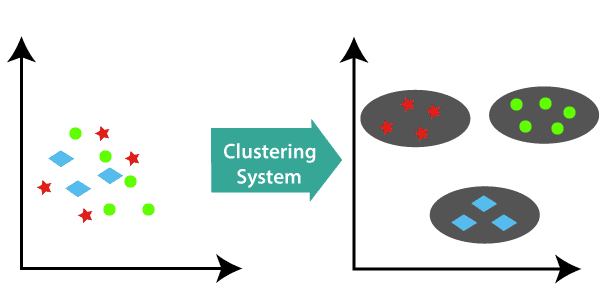# Although libraries like Scikit-Learn already help abstract the mathematical aspect of Machine learning model in practice, I think it is important as machine learning engineers and data science enthusiasts to concern ourselves with the mathematics behind the these algorithm.Salim Oyinlola

2 years ago | 4 min read

During my study of Machine Learning via Stanford University’s online platform, one area that particularly piqued my interest was unsupervised learning. I found it intriguing that a machine learning model could be trained such that it communicates with the features of a dataset without reference to any label. Unlike supervised learning where the algorithm is given a dataset in which the ‘right answers’ is given, unsupervised learning deals with modeling of the features of a dataset without reference to any label, and is often described as ‘allowing the dataset speak for itself’. Unsupervised learning is split into two: dimensionality reduction and clustering. Whilst the dimensionality reduction algorithm searches for more succinct representations of data, the clustering algorithm identifies distinct groups of data. These algorithms are concerned with learning from the properties of the data, an optimal division or discrete labeling of groups of points. In succeeding paragraphs, I would be dissecting the mathematics behind the simplest-to-understand clustering algorithm — K-Means clustering algorithm. This algorithm has various real-life uses that ranges from Recommender Systems to Vector Quantization to Cluster Analysis to Feature Learning to Bots and Anomaly Detection to name but a few. Although libraries like Scikit-Learn already help abstract the mathematical aspect of the model in practice, it is however important as machine learning engineers and data science enthusiasts to concern ourselves with the mathematics behind the ‘from sklearn.cluster import KMeans’K-Means Clustering is an unsupervised learning algorithm that is used to solve the clustering problems in machine learning or data science.

One important point to note about clusters created via a clustering algorithm is that the points within a cluster should be similar to each other. So, our aim here is to minimize the distance between the points within a cluster. K-means is a centroid-based algorithm, or a distance-based algorithm, where we calculate the distances to assign a point to a cluster. In K-Means, each cluster is associated with a centroid. The main objective of the algorithm is to minimize the sum of distances between the points and their respective cluster centroid.

The first step taken in k-means is to pick the number of clusters, k depending on the number of groups the data scientist wants to divide the data into. Thereafter, the algorithm randomly selects the centroid for each cluster. Let’s say we want to have 4 clusters, so k is equal to 4 here. It then randomly selects the centroid. Once it has successfully initialized the centroids using random initialization, it assigns each point to the closest cluster centroid by finding the Euclidean distance of each point in the dataset with the identified K points (cluster centroids). The algorithm then looks to find the new centroid by taking the average of the points in each cluster group. It is important to note that the euclidean distance is gotten using the formulae below.

The algorithm then repeats the process of finding the Euclidean distance of each point in the dataset with the identified K points (cluster centroids), assigning each data point to the closest centroid using the distance found in the previous step and finding the new centroid by taking the average of the points in each cluster group till one of the following occurs:

⦁ Centroids of newly formed clusters does not change

⦁ Points remains in the same cluster

⦁ Maximum number of iterations set by the data scientist working on the model is reached

After iteration is completed, the dataset takes the form shown below:

The algorithm then repeats the process of finding the Euclidean distance of each point in the dataset with the identified K points (cluster centroids), assigning each data point to the closest centroid using the distance found in the previous step and finding the new centroid by taking the average of the points in each cluster group till one of the following occurs:

⦁ Centroids of newly formed clusters does not change

⦁ Points remains in the same cluster

⦁ Maximum number of iterations set by the data scientist working on the model is reached

After iteration is completed, the dataset takes the form shown below:

Now, what are the major drawbacks of this algorithm? While this algorithm is relatively one of the better ones, one area that tends to affect its result is the number of clusters, k which is an input parameter. An inappropriate choice of k may yield poor results. That is why, when performing k-means, it is important to run diagnostic checks for determining the number of clusters in the data set. Also, although to a lesser degree, the convergence to a local minimum may produce counterintuitive (“wrong”) results. This implies that the result of k-means clustering might contradict the obvious cluster structure of the data set.

NOTE: In our implementation we chose the first 4 points as our initial cluster centroids which may give slightly different centroids each time the program is run on random dataset. We can also use the K-means++ method to choose our initial centroids. k-means++ was proposed in 2007 by Arthur and Vassilvitskii. This algorithm comes with a theoretical guarantee to find a solution that is O(log k) competitive to the optimal k-means solution. Sklearn KMeans class uses kmeans++ as the default method for seeding the algorithm.

UpvoteCreated by

Salim Oyinlola

Machine Learning and Artificial Intelligence enthusiastPost

Upvote

Downvote

Comment

Bookmark

Share

Related Articles